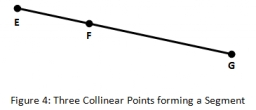# Length 20353

The given line is MN with a length of 11 cm. Change its length in the ratio:
a) k = 2: 1
b) k = 1: 2
c) k = 17: 11
d) k = 22: 33

a =  22 cm
b =  5.5 cm
c =  17 cm
d =  7.3333 cm

### Step-by-step explanation:Did you find an error or inaccuracy? Feel free to write us. Thank you!

Tips for related online calculators
Need help calculating sum, simplifying, or multiplying fractions? Try our fraction calculator.
Check out our ratio calculator.
Do you want to convert length units?